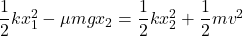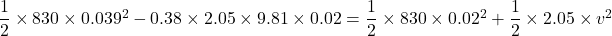## A 2.05 kg block on a horizontal floor is attached to a horizontal spring that is initially compressed 0.0390 m. The spring has force constan

Question

A 2.05 kg block on a horizontal floor is attached to a horizontal spring that is initially compressed 0.0390 m. The spring has force constant 830 N/m. The coefficient of kinetic friction between the floor and the block is 0.380. The block and spring are released from rest and the block slides along the floor.What is the speed of the block when it has moved a distance of 0.0200 m from its initial position?

in progress 0
2 months 2021-07-15T08:20:44+00:00 1 Answers 0 views 0

0.552 m/s

Explanation:

Given,

Mass of the block = 2.05 Kg

initial compression, x₁ = 0.03690 m

Spring constant, k = 830 N/m

coefficient of friction of the block, μ = 0.380

distance moved by the block,x₂ = 0.20 m

Speed, v  = ?

Using conservation of energy

Initial spring energy + Work done by friction = Final spring energy + kinetic energyv² = 0.3047

v = 0.552 m/s

Hence, the speed of the block is equal to 0.552 m/s